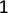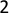Class Notes (1,100,000)
CA (650,000)
Queen's (10,000)
CHEM (300)
CHEM 112 (100)
Lecture 7

# CHEM 112 Lecture Notes - Lecture 7: Ideal Gas Law, Pressure Sensor, Ideal Gas

Department
Chemistry
Course Code
CHEM 112
Professor
Michael J Mombourquette
Lecture
7

This preview shows pages 1-3. to view the full 10 pages of the document.Experiment 7
1
Experiment 7. (1 week) (LGL) Gas Laws
Purpose
The purpose of this lab was to become comfortable with using the ideal gas law and testing the
relationship between pressure, volume, and temperature using experiments involving a pressure sensor
and a temperature probe.
Introduction1
In the 17th and 18th century, scientists began to notice that gases responded to changes in pressure,
temperature, and volume. These observations led to the development of a hypothesis then experiments
which eventually establish a now universal set of laws that govern the behavior of gases. These laws are
known as Boyle’s law, Charles’ law, Dalton’s law, and Avogadro’s law. Combined, these laws made one ideal
gas equation
PV=nRT
where P is pressure, V is volume, n is the number of moles, R is the gas constant, and t is the temperature in
Kelvin. This is the ideal gas law.
Ideal gases behave as though the gas molecules have no volume, and there are no intermolecular
forces between the molecules. There are no known gases that act precisely like this. However, in low
pressure and elevated temperature condition some gases behave nearly ideally. Gases like oxygen,
nitrogen, hydrogen and noble gases are considered to behave ideally at room temperature. This lab
assumes that all gasses are ideal and follow the ideal gas law. In this lab, all gases will be presumed to act
ideally and in accordance to the ideal gas law.
In part one of the lab, the relation between pressure and volume will be determined
experimentally. The gas will be air, and it will be confined in a syringe connected to a pressure sensor.
Changing the volume of gas in the syringe will cause a change in the pressure exerted on the gas. This will
be monitored on the computer. The relationship will then be analyzed. From the data, a mathematical
relationship will develop between pressure and volume of the confined gas. This is known as Boyle’s law.
In part two of the lab, the relationship between pressure and temperature is examined. The
pressure in a sealed flask is measured at varying temperatures. The gases are composed of particles that
are constantly in motion exerting pressure and colliding with the walls of the container. The velocity and
the number of collisions of these molecules affects the temperature and pressure that the gas exerts. Using
a Erlenmeyer flask containing an air sample in water bath (of varying temperature) the pressure and
temperature pairs will be collected and analyzed. From the data, a mathematical relationship will develop
between pressure and temperature of the confined gas.
In the final part of the lab, Dalton’s Laws will be used to determine the value of the gas constant. A
gas will be in a sealed container and the change in pressure will be measured. By knowing the number of
moles of gas added to the flask you will be able to determine the partial pressure of the gas. This will be the
gas constant.

Only pages 1-3 are available for preview. Some parts have been intentionally blurred.Experiment 7
2
Procedure1
Part 1
1. Open logger pro
2. Create the graph according to the instructions detailed in the lab manual
3. Prepare the syringe by moving the piston of the syringe until the front edge of the inner ring is at
the 10 mL mark
4. Attach the syringe to the pressure sensor
5. On logger pro click collect
6. Move the plunger so that the syringe is set at 5 mL and hold the plunger there until the pressure
stabilizes. After it stabilizes click keep and type 5.0 in the volume box. (repeat for 7.5, 10.0, 12.5,
15.0, 17.5, and 20.0 mL)
7. Click stop and record the pressure and volume data pairs
8. Determine the mathematical relationship of the graph by selecting curve fit in the analyze menu on
logger pro and trying the fit for linear and inverse functions
9. Take a screenshot of the graph
Part 2
1. Create a graph according to the instructions detailed in the lab manual
2. Prepare a rubber stopper flask assembly unit by tightly inserting the plastic pointed tips into each of
the openings on the rubber stopper.
3. Tightly insert the rubber stopper into the 125 mL Erlenmeyer flask
4. Connect the pressure sensor to one of the plastic tips using plastic tubing and add the valve switch
to the other tip
5. On logger pro click collect and ready the system for data collection of pressure and temperature at
4 temperatures (boiling, hot, room temperature, and in an ice water bath)
6. Immerse the flask in the boiling water bath and wait at least 2 minutes until the temperature
stabilizes. When the temperature is stable, close the valve. When both pressure and temperature
stabilize, click keep
7. Submerge the flask in the hot water bath until the pressure and temperature stabilize, then click
keep.
8. Repeat steps 6 and 7 for room temperature and ice water conditions
9. Convert the x axis to kelvin in logger pro
10. Set the parameters of the graph as outlined in the lab manual
11. Find the fit of the graph as outlined in step 8 of part 1 of this procedure
12. Take a screenshot of the graph

Only pages 1-3 are available for preview. Some parts have been intentionally blurred.Experiment 7
3
13. Take a screenshot of another graph using Celsius instead of kelvin
Part 3
1. Weigh 0.10g NaHCO3 into a clean 125 mL Erlenmeyer flask
2. Twist the two-holed stopper onto the flask
3. Fill the syringe with 1 M acetic acid
4. Attach the pressure sensor to one of the plastic tips on the stopper and place the assembly and
temperature probe into the room temperature bath
5. When the temperature stabilizes, record the pressure and temperature into the data sheet
6. Inject the acid into the flask then pull the plunger out to it’s original position
7. Swirl the flask until the pressure doesn’t rise
8. Record the final temperature and pressure
9. Determine the volume of the flask by filling the flask with water, then inserting the stopper back
into the flask to displace excess water, then use a graduated cylinder to measure the volume of the
Questions
Part 1
1. Based on your data, what would you expect the pressure to be if the volume of the syringe was
increased to 40.0 mL? Explain or show work to support your answer.
According to Boyle’s law, pressure and volume are inversely related. Thus, when the volume
doubled the pressure should have become half of that value.
When the volume is doubled, according to Boyle’s Law, pressure should be halved. So, our
experimental finding of 0.5253 atm would become 0.26265 atm.
2. Find the proportionality constant, k, from the data. If this relationship is direct P=kV, then k = P/V. If
it is inverse P=k/V, then k = P×V. Based on your data, Calculate k for both equations and then
indicate which formula gives the answer that is “constant” for all your P,V data pairs. Choose the
correct one of these formulas and calculate the average k value for the seven ordered pairs in your
data table (divide or multiply the P and V values). Calculate is the uncertainty, i.e. standard error, in
this final mean value of k? (Use statistics; see the section on determining this in the introduction
part of the lab manual)
 

             
 